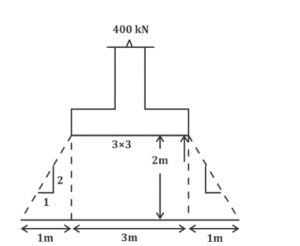Engineering Jobs   »   CIVIL ENGINEERING QUIZ

# RSMSSB-JE’21 CE: Daily Practices Quiz. 24-July-2021

Quiz: Civil Engineering
Exam: RSMSSB-JEn
Topic: SOIL MECHANICS

Each question carries 1 mark
Negative marking: 1/3 mark
Time: 8 Minutes

Q1. A soil has bulk density of 17.6 KN/m³ and water content 10%. if void ratio remains constant then the bulk density for water content of 20% will be
(a) 16.13 KN/m³
(b) 19.20 KN/m³
(c) 19.36 KN/m³
(d) 17.6 KN/m³

Q2. A fully saturated soil has a water content of 200 percent. If G = 2.6, the void ratio is:
(a) 1.3
(b) 2.6
(c) 5.2
(d) None of these above

Q3. A sand deposit has a porosity of 1/3 and its specific gravity is 2.5. The critical hydraulic gradient to cause sand boiling in the stratum will be
(a) 1.5
(b) 1.25
(c) 1.0
(d) 0.75

Q4. A load of 4000 kN is uniformly distributed over an area of 3m × 3m. Average vertical stress at a depth of 2m below will be (assuming 2 vertical : 1 horizontal distribution) –
(a) 250 kN/m²
(b) 160 kN/m²
(c) 200 kN/m²
(d) 220 kN/m²

Q5. Allowable bearing pressure for a foundation depends on:
(a) Allowable settlement only
(b) Ultimate bearing capacity only
(c) Both allowable settlement and ultimate bearing capacity
(d) Neither allowable settlement nor ultimate bearing capacity

Q6. Piles which are driven into the ground until a hard stratum is reached are termed as ________.
(a) friction piles
(b) bearing piles
(c) sheet piles
(d) batter piles

S1. Ans.(b)
Sol. 〖r_bulk〗_1=17.6 kN/m^3
W_1=10%
Void ratio (e)=constant
r_bulk2= ?>
w_2=20%
dry density (r_d )=r_b/(1+w)
r_b α (1+w)
〖r_b〗_1/〖r_b〗_2 =((1+w_1 ))/((1+w_2 ) )
17.6/〖r_b〗_2 =((1+0.1))/((1+0.2) )
▭(〖r_b〗_2=19.2)

S2. Ans.(c)
Sol. water content (w) = 200%
G= 2.6
Void Ratio (e) = ?
→ soil is fully saturated (S = 1)
Se = WG
1×e = 2×2.6
▭(e=5.2)

S3. Ans.(c)
Sol. porosity (n) = 1/3
G = 2.5
Critical hydraulic gradient (ic) = ?
e = n/(1-n )=(1\/3)/(2\/3)=0.5
▭(e=0.5)
i_c=(G-1)/(1+e)=(2.5-1)/(1+0.5)=1.5/1.5
▭(i_c=1 )

S4. Ans.(B)
Sol.Average vertical stress at a depth of 2 m below.
⇒ 4000/(5×5)
⇒160 kN\/m^2

S5. Ans.(c)
Sol. Allowable bearing pressure for a foundation depends on both allowable settlement and ultimate bearing capacity

S6. Ans.(b)
Sol. pile on the basis of their action –
End bearing pile – these piles are driven until a hard stratum is reached. These pile rest stiff or hard strata.
Friction pile – such piles are driven in soft clay or loose sand.

Sharing is caring!